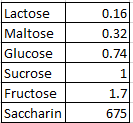### CAT 1999 Question 26

Instructions

DIRECTIONS for the following two questions: The following table presents the sweetness of different items relative to sucrose, whose sweetness is taken to be 1.00.Question 26

# What is the maximum amount of sucrose (to the nearest gram) that can be added to one-gram of saccharin such that the final mixture obtained is atleast 100 times as sweet as glucose?

Solution

For the mixture to be 100 times as sweet as glucose, its sweetness relative to the mixture should be at least 74.

1 gm of saccharin = 675

Let the number of grams of sucrose to be added be N. Thus, the total weight of the mixture = N + 1.

So, (675 + N) / (N+1) = 74

=> 675 + N = 74N + 74

=> 601 = 73N => N = 8.23

When N=9, sweetness will be S = (675+9)/10 = 684/10 = 68.4

When N=8, sweetness will be S = (675+8)/9 = 683/9 = 75.8

So, option b) is the correct answer.